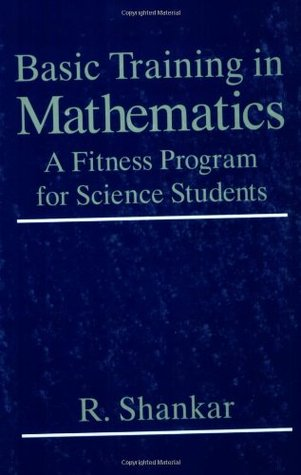# 耶鲁基础物理2.1. 复习匀加速运动\begin{equation} x(t) = x_0 + v_0t + \frac{1}{2} at^2 \label{2.1}\tag{2.1} \end{equation}

\begin{equation} v(t) = v_0 + at \label{2.2}\tag{2.2} \end{equation}

\begin{equation} t = \frac{v-v_0}{a} \label{2.3}\tag{2.3} \end{equation}

\begin{equation} v^2-v_0^2 = 2a(x-x_0) \label{2.4}\tag{2.4} \end{equation}Basic Training in Mathematics: A Fitness Program for Science Students

### 添加新评论